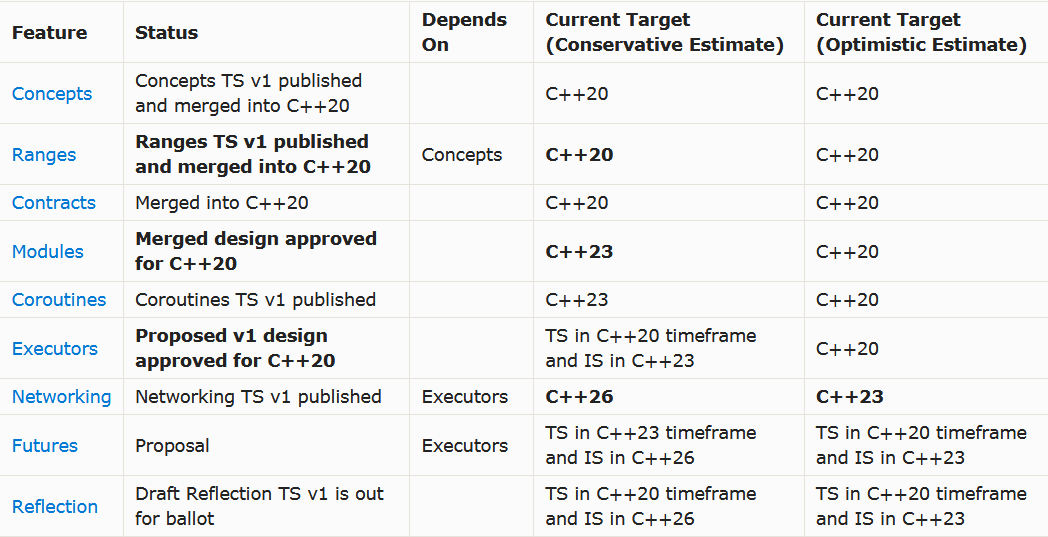# C++ 20 的悲叹，未出世就被群嘲“劝退”// A sample standard C++20 program that prints// the first N Pythagorean triples.#include <iostream>#include <optional>#include <ranges>   // New header! using namespace std; // maybe_view defines a view over zero or one// objects.template<Semiregular T>struct maybe_view : view_interface<maybe_view<T>> {  maybe_view() = default;  maybe_view(T t) : data_(std::move(t)) {  }  T const *begin() const noexcept {    return data_ ? &*data_ : nullptr;  }  T const *end() const noexcept {    return data_ ? &*data_ + 1 : nullptr;  }private:  optional<T> data_{};}; // "for_each" creates a new view by applying a// transformation to each element in an input// range, and flattening the resulting range of// ranges.// (This uses one syntax for constrained lambdas// in C++20.)inline constexpr auto for_each =  []<Range R,     Iterator I = iterator_t<R>,     IndirectUnaryInvocable<I> Fun>(R&& r, Fun fun)        requires Range<indirect_result_t<Fun, I>> {      return std::forward<R>(r)        | view::transform(std::move(fun))        | view::join;  }; // "yield_if" takes a bool and a value and// returns a view of zero or one elements.inline constexpr auto yield_if =  []<Semiregular T>(bool b, T x) {    return b ? maybe_view{std::move(x)}             : maybe_view<T>{};  }; int main() {  // Define an infinite range of all the  // Pythagorean triples:  using view::iota;  auto triples =    for_each(iota(1),  {      return for_each(iota(1, z+1), = {        return for_each(iota(x, z+1), = {          return yield_if(x*x + y*y == z*z,            make_tuple(x, y, z));        });      });    });    // Display the first 10 triples    for(auto triple : triples | view::take(10)) {      cout << '('           << get<0>(triple) << ','           << get<1>(triple) << ','           << get<2>(triple) << ')' << '\n';  }}

void printNTriples(int n){    int i = 0;    for (int z = 1; ; ++z)        for (int x = 1; x <= z; ++x)            for (int y = x; y <= z; ++y)                if (x*x + y*y == z*z) {                    printf("%d, %d, %d\n", x, y, z);                    if (++i == n)                        return;                }}

// simplest.cpp#include <time.h>#include <stdio.h>int main(){    clock_t t0 = clock();     int i = 0;    for (int z = 1; ; ++z)        for (int x = 1; x <= z; ++x)            for (int y = x; y <= z; ++y)                if (x*x + y*y == z*z) {                    printf("(%i,%i,%i)\n", x, y, z);                    if (++i == 100)                        goto done;                }    done:     clock_t t1 = clock();    printf("%ims\n", (int)(t1-t0)*1000/CLOCKS_PER_SEC);    return 0;}

(3,4,5)(6,8,10)(5,12,13)(9,12,15)(8,15,17)(12,16,20)(7,24,25)(15,20,25)(10,24,26)...(65,156,169)(119,120,169)(26,168,170)

// simple-reusable.cpp#include <time.h>#include <stdio.h>struct pytriples{    pytriples() : x(1), y(1), z(1) {}    void next()    {        do        {            if (y <= z)                ++y;            else            {                if (x <= z)                    ++x;                else                {                    x = 1;                    ++z;                }                y = x;            }        } while (x*x + y*y != z*z);    }    int x, y, z;};int main(){    clock_t t0 = clock();     pytriples py;    for (int c = 0; c < 100; ++c)    {        py.next();        printf("(%i,%i,%i)\n", py.x, py.y, py.z);    }     clock_t t1 = clock();    printf("%ims\n", (int)(t1-t0)*1000/CLOCKS_PER_SEC);    return 0;} 

generator<std::tuple<int,int,int>> pytriples(){    for (int z = 1; ; ++z)        for (int x = 1; x <= z; ++x)            for (int y = x; y <= z; ++y)                if (x*x + y*y == z*z)                    co_yield std::make_tuple(x, y, z);}

C ++20 Ranges 会让整段代码更加清晰吗？结果如下：

auto triples =    for_each(iota(1),  {        return for_each(iota(1, z+1), = {            return for_each(iota(x, z+1), = {                return yield_if(x*x + y*y == z*z,                    make_tuple(x, y, z));                });            });        });

## C++ 存在的问题有哪些？

// ranges.cpp#include <time.h>#include <stdio.h>#include <range/v3/all.hpp>using namespace ranges;int main(){    clock_t t0 = clock();     auto triples = view::for_each(view::ints(1),  {        return view::for_each(view::ints(1, z + 1), = {            return view::for_each(view::ints(x, z + 1), = {                return yield_if(x * x + y * y == z * z,                    std::make_tuple(x, y, z));            });        });    });     RANGES_FOR(auto triple, triples | view::take(100))    {        printf("(%i,%i,%i)\n", std::get<0>(triple), std::get<1>(triple), std::get<2>(triple));    }     clock_t t1 = clock();    printf("%ims\n", (int)(t1-t0)*1000/CLOCKS_PER_SEC);    return 0;}

C++20 比简单版本的代码编译时间长近 3 秒

range-v3 是 1.8 兆字节的源代码，全部在头文件中，因此，虽然使用 C++ 20 输出 100 个三元数组的代码示例只有 30 行，但加上头文件后，编译器最终会编译 102,000 行代码。在所有预处理之后，简单版本的 C ++ 示例只有 720 行代码。

Ranges 示例的运行时性能慢了 150 倍，这对于要解决实际问题的代码库而言，两个数量级的速度可能意味着不会对任何实际数据集起作用。该开发者在游戏行业工作，这意味着引擎或工具的 Debug 版本不适用于任何真实的游戏级别模拟（性能无法接近所需的交互级别）。

## 其他语言如何？

using System;using System.Diagnostics;using System.Linq;class Program{    public static void Main()    {        var timer = Stopwatch.StartNew();        var triples =            from z in Enumerable.Range(1, int.MaxValue)            from x in Enumerable.Range(1, z)            from y in Enumerable.Range(x, z)            where x*x+y*y==z*z            select (x:x, y:y, z:z);        foreach (var t in triples.Take(100))        {            Console.WriteLine($"({t.x},{t.y},{t.z})"); } timer.Stop(); Console.WriteLine($"{timer.ElapsedMilliseconds}ms");    }}

var triples =    from z in Enumerable.Range(1, int.MaxValue)    from x in Enumerable.Range(1, z)    from y in Enumerable.Range(x, z)    where x*x+y*y==z*z    select (x:x, y:y, z:z);

auto triples = view::for_each(view::ints(1),  {    return view::for_each(view::ints(1, z + 1), = {        return view::for_each(view::ints(x, z + 1), = {            return yield_if(x * x + y * y == z * z,                std::make_tuple(x, y, z));        });    });});

C＃LINQ 的另一种“数据库较少”的形式：

var triples = Enumerable.Range(1, int.MaxValue)    .SelectMany(z => Enumerable.Range(1, z), (z, x) => new {z, x})    .SelectMany(t => Enumerable.Range(t.x, t.z), (t, y) => new {t, y})    .Where(t => t.t.x * t.t.x + t.y * t.y == t.t.z * t.t.z)    .Select(t => (x: t.t.x, y: t.y, z: t.t.z));

## 为什么 C ++ 会这样？